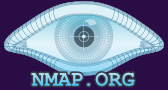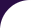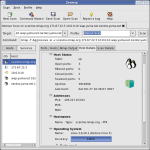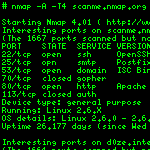Intro Reference Guide Book Install Guide Download Changelog Zenmap GUI Docs Bug Reports OS Detection Propaganda Related Projects In the Movies In the News## Understanding an Nmap Fingerprint

When Nmap stores a fingerprint in memory, Nmap uses a tree of attributes and values in data structures that users need not even be aware of. But there is also a special ASCII-encoded version which Nmap can print for users when a machine is unidentified. Thousands of these serialized fingerprints are also read back every time Nmap runs (with OS detection enabled) from the `nmap-os-db` database. The fingerprint format is a compromise between human comprehension and brevity. The format is so terse that it looks like line noise to many inexperienced users, but those who read this document should be able to decipher fingerprints with ease. There are actually two types of fingerprints, though they have the same general structure. The fingerprints of known operating systems that Nmap reads in are called reference fingerprints, while the fingerprint Nmap displays after scanning a system is a subject fingerprint. The reference fingerprints are a bit more complex since they can be tailored to match a whole class of operating systems by adding leeway to (or omitting) tests that aren't so reliable while allowing only a single possible value for other tests. The reference fingerprints also have OS details and classifications. Since the subject tests are simpler, we describe them first.

### Decoding the Subject Fingerprint Format

If Nmap performs OS fingerprinting on a host and doesn't get a perfect OS matches despite promising conditions (such as finding both open and closed ports accessible on the target), Nmap prints a subject fingerprint that shows all of the test results that Nmap deems relevant, then asks the user to submit the data to Nmap.Org. Tests aren't shown when Nmap has no useful results, such as when the relevant probe responses weren't received. A special line named `SCAN` gives extra details about the scan (such as Nmap version number) that provide useful context for integrating fingerprint submissions into `nmap-os-db`. A typical subject fingerprint is shown in Example 8.3.

Example 8.3. A typical subject fingerprint

```OS:SCAN(V=5.05BETA1%D=8/23%OT=22%CT=1%CU=42341%PV=N%DS=0%DC=L%G=Y%TM=4A91CB
OS:90%P=i686-pc-linux-gnu)SEQ(SP=C9%GCD=1%ISR=CF%TI=Z%CI=Z%II=I%TS=A)OPS(O1
OS:=M400CST11NW5%O2=M400CST11NW5%O3=M400CNNT11NW5%O4=M400CST11NW5%O5=M400CS
OS:T11NW5%O6=M400CST11)WIN(W1=8000%W2=8000%W3=8000%W4=8000%W5=8000%W6=8000)
OS:ECN(R=Y%DF=Y%T=40%W=8018%O=M400CNNSNW5%CC=N%Q=)T1(R=Y%DF=Y%T=40%S=O%A=S+
OS:%F=AS%RD=0%Q=)T2(R=N)T3(R=Y%DF=Y%T=40%W=8000%S=O%A=S+%F=AS%O=M400CST11NW
OS:5%RD=0%Q=)T4(R=Y%DF=Y%T=40%W=0%S=A%A=Z%F=R%O=%RD=0%Q=)T5(R=Y%DF=Y%T=40%W
OS:=0%S=Z%A=S+%F=AR%O=%RD=0%Q=)T6(R=Y%DF=Y%T=40%W=0%S=A%A=Z%F=R%O=%RD=0%Q=)
OS:T7(R=Y%DF=Y%T=40%W=0%S=Z%A=S+%F=AR%O=%RD=0%Q=)U1(R=Y%DF=N%T=40%IPL=164%U
OS:N=0%RIPL=G%RID=G%RIPCK=G%RUCK=G%RUD=G)IE(R=Y%DFI=N%T=40%CD=S)
```

Now you may look at this fingerprint and immediately understand what everything means. If so, you can simply skip this section. But I have never seen such a reaction. Many people probably think some sort of buffer overflow or unterminated string error is causing Nmap to spew garbage data at them. This section helps you decode the information so you can immediately tell that blind TCP sequence prediction attacks against this machine are moderately hard, but it may make a good idle scan (`-sI`) zombie. The first step in understanding this fingerprint is to fix the line wrapping. The tests are all squished together, with each line wrapped at 71 characters. Then `OS:` is prepended to each line, raising the length to 74 characters. This makes fingerprints easy to cut and paste into the Nmap fingerprint submission form (see the section called “When Nmap Fails to Find a Match and Prints a Fingerprint”). Removing the prefix and fixing the word wrapping (each line should end with a right parenthesis) leads to the cleaned-up version in Example 8.4.

Example 8.4. A cleaned-up subject fingerprint

```SCAN(V=5.05BETA1%D=8/23%OT=22%CT=1%CU=42341%PV=N%DS=0%DC=L%G=Y%TM=4A91CB90%
P=i686-pc-linux-gnu)
SEQ(SP=C9%GCD=1%ISR=CF%TI=Z%CI=Z%II=I%TS=A)
OPS(O1=M400CST11NW5%O2=M400CST11NW5%O3=M400CNNT11NW5%
O4=M400CST11NW5%O5=M400CST11NW5%O6=M400CST11)
WIN(W1=8000%W2=8000%W3=8000%W4=8000%W5=8000%W6=8000)
ECN(R=Y%DF=Y%T=40%W=8018%O=M400CNNSNW5%CC=N%Q=)
T1(R=Y%DF=Y%T=40%S=O%A=S+%F=AS%RD=0%Q=)
T2(R=N)
T3(R=Y%DF=Y%T=40%W=8000%S=O%A=S+%F=AS%O=M400CST11NW5%RD=0%Q=)
T4(R=Y%DF=Y%T=40%W=0%S=A%A=Z%F=R%O=%RD=0%Q=)
T5(R=Y%DF=Y%T=40%W=0%S=Z%A=S+%F=AR%O=%RD=0%Q=)
T6(R=Y%DF=Y%T=40%W=0%S=A%A=Z%F=R%O=%RD=0%Q=)
T7(R=Y%DF=Y%T=40%W=0%S=Z%A=S+%F=AR%O=%RD=0%Q=)
U1(R=Y%DF=N%T=40%IPL=164%UN=0%RIPL=G%RID=G%RIPCK=G%RUCK=G%RUD=G)
IE(R=Y%DFI=N%T=40%CD=S)
```

While this still isn't the world's most intuitive format (we had to keep it short), the format is much clearer now. Every line is a category, such as `SEQ` for the sequence generation tests, `T3` for the results from that particular TCP probe, and `IE` for tests related to the two ICMP echo probes.

Following each test name is a pair of parentheses which enclose results for individual tests. The tests take the format `<testname>=<value>`. All of the possible categories, tests, and values are described in the section called “TCP/IP Fingerprinting Methods Supported by Nmap”. Each pair of tests are separated by a percentage symbol (%). Tests values can be empty, leading to a percentage symbol or category-terminating right-parenthesis immediately following the equal sign. The string `O=%RD=0%Q=)` in `T4` of our example shows two of these empty tests. A blank test value must match another blank value, so this empty TCP quirks `Q` value wouldn't match a fingerprint with `Q` set to `RU`.

In some cases, a whole test is missing rather than just its value. For example, `T2` of our sample fingerprint has no `W` (TCP window), `S` (sequence number), `A` (acknowledgment number), `T` (TTL), or `TG` (TTL guess) tests. This is because the one test and value it does include, `R=N`, means that no response was returned for the `T2` probe. So including a window value or sequence number would make little sense. Similarly, tests which aren't well supported on the system running Nmap are skipped. An example is the `RID` (IP ID field returned in ICMP packet) test, which doesn't work well on Solaris because that system tends to corrupt the `ID` field Nmap sends out. Tests which are inconclusive (such as failing to detect the IP ID sequence for the `TI`, `CI`, and `II` tests) are also omitted.

#### Decoding the `SCAN` line of a subject fingerprint

The `SCAN` line is a special case in a subject fingerprint. Rather than describe the target system, these tests describe various conditions of the scan. These help us integrate fingerprints submitted to Nmap.Org. The tests in this line are:

• Nmap version number (`V`).

• Date of scan (`D`) in the form month/day.

• Open and closed TCP ports (on target) used for scan (`OT` and `CT`). Unlike most tests, these are printed in decimal format. If Nmap was unable to find an open or a closed port, the test is included with an empty value (even when Nmap guesses a possibly closed port and sends a probe there).

• Closed UDP port (`CU`). This is the same as `CT`, but for UDP. Since the majority of scans don't include UDP, this test's value is usually empty.

• Private IP space (`PV`) is `Y` if the target is on the `10.0.0.0/8`, `172.16.0.0/12`, or `192.168.0.0/16` private networks (RFC 1918). Otherwise it is `N`.

• Network distance (`DS`) is the network hop distance from the target. It is `0` if the target is localhost, `1` if directly connected on an ethernet network, or the exact distance if discovered by Nmap. If the distance is unknown, this test is omitted.

• The distance calculation method (`DC`) indicates how the network distance (`DS`) was calculated. It can take on these values: `L` for localhost (`DS=0`); `D` for a direct subnet connection (`DS=1`); `I` for a TTL calculation based on an ICMP response to the `U1` OS detection probe; and `T` for a count of traceroute hops. This test exists because it is possible for the ICMP TTL calculation to be incorrect when intermediate machines change the TTL; it distinguishes between a host that is truly directly connected and what may be just a miscalculation.

• Good results (`G`) is `Y` if conditions and results seem good enough to submit this fingerprint to Nmap.Org. It is `N` otherwise. Unless you force them by enabling debugging (`-d`) or extreme verbosity (`-vv`), `G=N` fingerprints aren't printed by Nmap.

• Target MAC prefix (`M`) is the first six hex digits of the target MAC address, which correspond to the vendor name. Leading zeros are not included. This field is omitted unless the target is on the same ethernet network (`DS=1`).

• The OS scan time (`TM`) is provided in Unix time_t format (in hexadecimal).

• The platform Nmap was compiled for is given in the `P` field.

### Decoding the Reference Fingerprint Format

When Nmap scans a target to create a subject fingerprint, it then tries to match that data against the thousands of reference fingerprints in the `nmap-os-db` database. Reference fingerprints are initially formed from one or more subject fingerprints and thus have much in common. They do have a bit of extra information to facilitate matching and of course to describe the operating systems they represent. For example, the subject fingerprint we just looked at might form the basis for the reference fingerprint in Example 8.5.

Example 8.5. A typical reference fingerprint

```Fingerprint Apple Mac OS X Server 10.2.8 (Jaguar) (Darwin 6.8, PowerPC)
Class Apple | Mac OS X | 10.2.X | general purpose
CPE cpe:/o:apple:mac_os_x:10.2.8
SEQ(SP=FB-111%GCD=1-6%ISR=104-10E%TI=I%II=I%SS=S%TS=1)
OPS(O1=M5B4NW0NNT11%O2=M5B4NW0NNT11%O3=M5B4NW0NNT11%O4=M5B4NW0NNT11%O5=M5B4NW0NNT11%O6=M5B4NNT11)
WIN(W1=8218%W2=8220%W3=8204%W4=80E8%W5=80F4%W6=807A)
ECN(R=Y%DF=Y%T=3B-45%TG=40%W=832C%O=M5B4NW0%CC=N%Q=)
T1(R=Y%DF=Y%T=3B-45%TG=40%S=O%A=S+%F=AS%RD=0%Q=)
T2(R=N)
T3(R=Y%DF=Y%T=3B-45%TG=40%W=807A%S=O%A=S+%F=AS%O=M5B4NW0NNT11%RD=0%Q=)
T4(R=Y%DF=Y%T=3B-45%TG=40%W=0%S=A%A=Z%F=R%O=%RD=0%Q=)
T5(R=Y%DF=N%T=3B-45%TG=40%W=0%S=Z%A=S+%F=AR%O=%RD=0%Q=)
T6(R=Y%DF=Y%T=3B-45%TG=40%W=0%S=A%A=Z%F=R%O=%RD=0%Q=)
T7(R=Y%DF=N%T=3B-45%TG=40%W=0%S=Z%A=S%F=AR%O=%RD=0%Q=)
U1(DF=N%T=3B-45%TG=40%IPL=38%UN=0%RIPL=G%RID=G%RIPCK=G%RUCK=0%RUD=G)
IE(DFI=S%T=3B-45%TG=40%CD=S)
```

Some differences are immediately obvious. Line wrapping is not done because that is only important for the submission process. The `SCAN` line is also removed, since that information describes a specific scan instance rather than general target OS characteristics.

You probably also noticed the new lines, `Fingerprint`, `Class`, and `CPE`, which are new to this reference fingerprint. A more subtle change is that some of the individual test results have been removed while others have been enhanced with logical expressions.

#### Free-form OS description (`Fingerprint` line)

The `Fingerprint` line first serves as a token so Nmap knows to start loading a new fingerprint. Each fingerprint only has one such line. Immediately after the `Fingerprint` token (and a space) comes a textual description of the operating system(s) represented by this fingerprint. These are in free-form English text, designed for human interpretation rather than a machine parser. Nevertheless, Nmap tries to stick with a consistent format including the vendor, product name, and then version number. Version number ranges and comma-separated alternatives discussed previously can be found in this field. Here are some examples:

```Fingerprint HP LaserJet printer (4050, 4100, 4200, or 8150)
Fingerprint Sun Solaris 9 or 10 (SPARC)
Fingerprint Linux 2.6.22 - 2.6.24
Fingerprint Microsoft Windows Server 2003 SP1
Fingerprint Microsoft Windows XP Professional SP1
Fingerprint Minolta Di550 laser printer
```

In an ideal world, every different OS would correspond to exactly one unique fingerprint. Unfortunately, OS vendors don't make life so easy for us. The same OS release may fingerprint differently based on what network drivers are in use, user-configurable options, patch levels, processor architecture, amount of RAM available, firewall settings, and more. Sometimes the fingerprints differ for no discernible reason. While the reference fingerprint format has an expression syntax for coping with slight variations, creating multiple fingerprints for the same OS is often preferable when major differences are discovered.

Just as multiple fingerprints are often needed for one OS, sometimes a single fingerprint describes several systems. If two systems give the exact same results for every single test, Nmap has little choice but to offer up both as possibilities. This commonly occurs for several reasons. One is that vendors may release a new version of their OS without any significant changes to their IP stack. Maybe they made important changes elsewhere in the system, or perhaps they did little but want to make a bunch of money selling upgrades. In these cases, Nmap often prints a range such as `Apple Mac OS X 10.4.8 - 10.4.11` or `Sun Solaris 9 or 10`.

Another cause of duplicate fingerprints is embedded devices which share a common OS. For example, a printer from one vendor and an ethernet switch from another may actually share an embedded OS from a third vendor. In many cases, subtle differences between the devices still allow them to be distinguished. But sometimes Nmap must simply list a group of possibilities such as ```Cisco 1200-series WAP, HP ProCurve 2650 switch, or Xerox Phaser 7400N or 8550DT printer```.

There are also cases where numerous vendors private label the exact same OEM device with their own brand name and model number. Here again, Nmap must simply list the possibilities. But distinguishing these is less important because they are all fundamentally the same device.

TipIf the description printed by Nmap (which comes from the `Fingerprint` line) isn't informative enough for you, more detailed information may be available in comments above the fingerprint itself in `nmap-os-db`. You can find it installed on your system as described in Chapter 14, Understanding and Customizing Nmap Data Files, or look up the latest version at https://svn.nmap.org/nmap/nmap-os-db. Search for the exact OS description that Nmap gives you. Keep in mind that there may be several `Fingerprint` lines with exactly the same description, so you may have to examine them all. Or use the Nmap XML output, which shows the line number of each match.

#### Device and OS classification (`Class` lines)

While the `Fingerprint` description works great for analysts reading Nmap output directly, many people run Nmap from other scripts and applications. Those applications might use the OS information to check for OS-specific vulnerabilities or just create a pretty graph or report.

A more structured OS classification system exists for these purposes. It is also useful when there are multiple matches. If you only get a partial fingerprint (maybe no open ports were found on the target so many tests had to be skipped), it might match dozens of different fingerprints in the `nmap-os-db` database. Printing the details for all of those fingerprints would be a mess. But thanks to OS classification, Nmap can find commonality. If all of the matches are classified as Linux, Nmap will simply print that the target is a Linux box.

Every fingerprint has one or more `Class` lines. Each contains four well-defined fields: vendor, OS family, OS generation, and device type. The fields are separated by the pipe symbol (`|`).

The vendor is the company which makes an OS or device. Examples are `Apple`, `Cisco`, `Microsoft`, and `Linksys`. For community projects such as OpenBSD and Linux without a controlling vendor, the OS family name is repeated for the vendor column.

OS family includes products such as `Windows`, `Linux`, `IOS` (for Cisco routers), `Solaris`, and `OpenBSD`. There are also hundreds of devices such as switches, broadband routers, and printers which use undisclosed operating systems. When the underlying OS isn't clear, `embedded` is used.

OS generation is a more granular description of the OS. Generations of Linux include `2.4.X` and `2.6.X`, while Windows generations include `95`, `98`, `Me`, `2000`, `XP`, and `Vista`. FreeBSD uses generations such as `4.X` and `5.X`. For obscure operating systems which we haven't subdivided into generations (or whenever the OS is listed simply as `embedded`), this field is left blank.

The device type is a broad classification such as `router`, `printer`, or `game console` and was discussed previously in this chapter. General-purpose operating systems such as `Linux` and `Windows` which can be used for just about anything are classified as `general purpose`.

Each field may contain just one value. When a fingerprint represents more than one possible combination of these four fields, multiple `Class` lines are used. Example 8.6 provides some example `Fingerprint` lines followed by their corresponding classifications.

Example 8.6. Some typical fingerprint descriptions and corresponding classifications

```Fingerprint D-Link DSL-500G ADSL router

Fingerprint Linksys WRT54GC or TRENDnet TEW-431BRP WAP
Class Linksys | embedded || WAP
Class TRENDnet | embedded || WAP

Fingerprint Apple Mac OS X 10.3.9 (Panther) - 10.4.7 (Tiger)
Class Apple | Mac OS X | 10.3.X | general purpose
Class Apple | Mac OS X | 10.4.X | general purpose

Fingerprint Sony PlayStation 3 game console
Class Sony | embedded || game console
```

If these examples aren't enough, a listing of classifications recognized by the latest version of Nmap is maintained at http://nmap.org/data/os-classes.txt.

#### CPE name (`CPE` lines)

`CPE` lines give Common Platform Enumeration equivalents of `Class` lines. Each `Class` may be followed by several `CPE` lines (`CPE` lines always belong to the `Class` line that immediately precedes them). It's common for one CPE name to describe the operating system and another to describe the hardware platform. A description of CPE syntax and meaning can be found in the section called “Common Platform Enumeration (CPE)”.

Example 8.7. Typical CPE classifications

```Fingerprint 3Com NBX 100 VoIP gateway (VxWorks)
Class 3Com | VxWorks || VoIP adapter
CPE cpe:/h:3com:nbx_100
CPE cpe:/o:3com:vxworks auto

Fingerprint Linux 2.0.33
Class Linux | Linux | 2.0.X | general purpose
CPE cpe:/o:linux:linux_kernel:2.0.33
```

The `auto` flag that follows some CPE names is not part of CPE; it is only used internally by maintenance scripts to indicate that a CPE name was automatically generated from other information rather than inserted manually.

#### Test expressions

The test expressions don't have to change between a subject and reference fingerprint, but they almost always do. The reference fingerprint often needs to be generalized a little bit to match all instances of a particular OS, rather than just the machine you are scanning. For example, some Windows XP machines return a Window size of `F424` to the `T1` probe, while others return `FAF0`. This may be due to the particular ethernet device driver in use, or maybe how much memory is available. In any case, we would like to detect Windows XP no matter which window size is used.

One way to generalize a fingerprint is to simply remove tests that produce inconsistent results. Remove all of the window size tests from a reference fingerprint, and systems will match that print no matter what size they use. The downside is that you can lose a lot of important information this way. If the only Window sizes that a particular system ever sends are `F424` and `FAF0`, you really only want to allow those two values, not all 65,536 possibilities.

While removing tests is overkill in some situations, it is useful in others. The `R=Y` test value, meaning there was a response, is usually removed from the `U1` and `IE` tests before they are added to `nmap-os-db`. These probes are often blocked by a firewall, so the lack of a response should not count against the OS match.

When removing tests is undesirable, Nmap offers an expression syntax for allowing a test to match multiple values. For example, `W=F424|FAF0` would allow those two Windows XP window values without allowing any others. Table 8.8 shows the permitted operators in test values.

Table 8.8. Reference fingerprint test expression operators

Op NameSymbolExampleDescription
Or`|``O=|ME|MNNTNW`Matches if the corresponding subject fingerprint test takes the value of any of the clauses. In this example, the initial pipe symbol means that an empty options list will match too.
Range`-``SP=7-A`Matches if the subject fingerprint's corresponding test produces a numeric value which falls within the range specified.
Greater than`>``SP=>8`Matches if the subject fingerprint's corresponding test produces a numeric value which is greater than the one specified.
Less than`<``GCD=<5`Matches if the subject fingerprint's corresponding test produces a numeric value which is less than the one specified.

Expressions can combine operators, as in `GCD=1-6|64|256|>1024`, which matches if the `GCD` is between one and six, exactly 64, exactly 256, or greater than 1024.

### IPv6 fingerprints

Because the IPv6 classification engine works differently, it has different fingerprints. There are no reference fingerprints; instead a set of previously identified training examples is run through a training algorithm, which outputs a large matrix of coefficients, one for each feature and OS class. Subject fingerprints use the same ASCII-armored format as IPv4, as shown in Example 8.8, “An IPv6 fingerprint”.

Example 8.8. An IPv6 fingerprint

```OS:SCAN(V=5.61TEST1%E=6%D=9/27%OT=22%CT=443%CU=42192%PV=N%DS=5%DC=T%G=Y%TM
OS:=4E82908D%P=x86_64-unknown-linux-gnu)S1(P=6000{4}28063cXX{32}0016c1b002
OS:bbd213c57562f5a01212e0f8880000020404c40402080a5be177f2ff{4}01030307%ST=
OS:0.021271%RT=0.041661)S2(P=6000{4}28063cXX{32}0016c1b108d7da47c57562f6a0
OS:1212e0e9d20000020404c40402080a5be17856ff{4}01030307%ST=0.121251%RT=0.14
OS:4586)S3(P=6000{4}28063cXX{32}0016c1b21029efebc57562f7a01212e0cf63000002
OS:0404c40101080a5be178ceff{4}01030307%ST=0.221232%RT=0.268086)S4(P=6000{4
OS:}28063cXX{32}0016c1b31553d32dc57562f8a01212e0e3a40000020404c40402080a5b
OS:e1791eff{4}01030307%ST=0.321237%RT=0.340261)S5(P=6000{4}28063cXX{32}001
OS:6c1b41ae90087c57562f9a01212e0b04f0000020404c40402080a5be17982ff{4}01030
OS:307%ST=0.421246%RT=0.441253)S6(P=6000{4}24063cXX{32}0016c1b5207baa83c57
OS:562fa901212e014690000020404c40402080a5be179e6ff{4}%ST=0.521245%RT=0.541
OS:755)IE1(P=6000{4}803a3cXX{32}810927cbabcd00{122}%ST=0.565533%RT=0.59350
OS:5)U1(P=6000{3}01643a3cXX{32}0104be5300{4}6001234501341131XX{32}c1a9a4d0
OS:013482ff43{300}%ST=0.713832%RT=0.734263)TECN(P=6000{4}20063cXX{32}0016c
OS:1b62f0c74d8c57562fb80121310241c0000020404c40101040201030307%ST=0.763567
OS:%RT=0.784838)T2(P=6000{4}583a3cXX{32}0101ca5600{4}6001234500280632XX{32
OS:}c1b70016c57562fb85549cefa00000808c0d000003030a0102040109080aff{4}00{4}
OS:0402%ST=0.813012%RT=0.833344)T3(P=6000{4}583a3cXX{32}0101ca6000{4}60012
OS:34500280628XX{32}c1b80016c57562fc2b8e3db7a02b0100445f000003030a01020401
OS:09080aff{4}00{4}0402%ST=0.863293%RT=0.881198)T4(P=6000{4}14063cXX{32}00
OS:16c1b93a67fc8a00{4}500400000c7c0000%ST=0.912394%RT=0.93247)T5(P=6000{4}
OS:14063cXX{32}01bbc1ba00{4}c57562ff5014000019430000%ST=0.96164%RT=0.98347
OS:5)T6(P=6000{4}14063cXX{32}01bbc1bba9e336d500{4}50040000610e0000%ST=1.01
OS:164%RT=1.03554)T7(P=6000{4}583a3cXX{32}0101ca5800{4}6001234500280630XX{
OS:32}c1bc01bbc5756300095eb241a029ffffec59000003030f0102040109080aff{4}00{
OS:4}0402%ST=1.06173%RT=1.07961)EXTRA(FL=12345)
```

Example 8.9, “A cleaned-up IPv6 fingerprint” shows what this fingerprint looks like unwrapped. Most of the probes are omitted because they all have the same format.

Example 8.9. A cleaned-up IPv6 fingerprint

```SCAN(V=5.61TEST1%E=6%D=9/27%OT=22%CT=443%CU=42192%PV=N%DS=5%DC=T%G=Y%TM4E82908D%
P=x86_64-unknown-linux-gnu)
S1(P=6000{4}28063cXX{32}0016c1b002bbd213c57562f5a01212e0f8880000020404c4040208
0a5be177f2ff{4}01030307%ST=0.021271%RT=0.041661)
EXTRA(FL=12345)
```

The `SCAN` line has the same meaning as in IPv4 fingerprints. The pseudo-test `E=6` indicates that this is an IPv6 fingerprint.

Then there is one line for each probe that received a response. Within each of these there are three keys:

`P`

The contents of the packet, hex- and run-length–encoded. Whenever two digits are followed by a number in curly brackets, it means to repeat that byte the given number of times. For example, `00{4}` is short for `00000000`. The characters `XX` are put in place of source and destination addresses, which are private and anyway not useful for training the classifier.

`ST`

The time when the packet was sent, in seconds, relative to when OS detection began.

`RT`

The time when the packet was received.

The `EXTRA` line stores any other information that can't be determined purely from the packet contents. The `FL` key stores the flow label that was sent during the scan. This would always be `12345`, except that some operating systems don't allow setting the flow label and always send `00000` instead.

When a fingerprint like the one above is processed, it is converted to an internal representation as shown below. Each value is one element of the feature vector that is passed to the classifier. They correspond to the features listed in the section called “List of all features”.

```        40  S1.PLEN
0  S1.TC
40  S2.PLEN
0  S2.TC
40  S3.PLEN
0  S3.TC
40  S4.PLEN
0  S4.TC
40  S5.PLEN
0  S5.TC
36  S6.PLEN
0  S6.TC
128  IE1.PLEN
0  IE1.TC
UNKNOWN  IE2.PLEN
UNKNOWN  IE2.TC
UNKNOWN  NS.PLEN
UNKNOWN  NS.TC
356  U1.PLEN
0  U1.TC
`<Many more lines omitted.>`
```

`UNKNOWN` values usually mean that no response was received to the relevant probe. `UNKNOWN` values are mapped to −1 before classification.

[ Nmap | Sec Tools | Mailing Lists | Site News | About/Contact | Advertising | Privacy ]